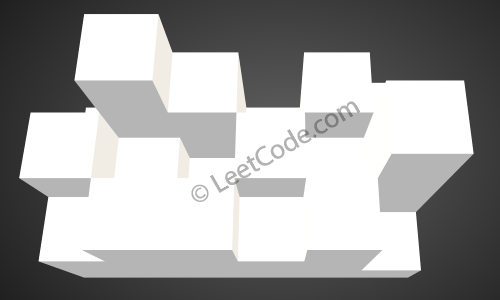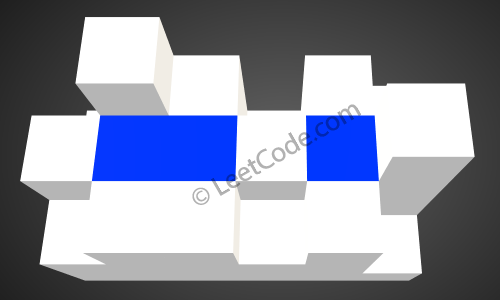##### Welcome to Subscribe On Youtube

Formatted question description: https://leetcode.ca/all/407.html

# 407. Trapping Rain Water II

Hard

## Description

Given an m x n matrix of positive integers representing the height of each unit cell in a 2D elevation map, compute the volume of water it is able to trap after raining.

Note:

Both m and n are less than 110. The height of each unit cell is greater than 0 and is less than 20,000.

Example:

Given the following 3x6 height map:
[
[1,4,3,1,3,2],
[3,2,1,3,2,4],
[2,3,3,2,3,1]
]

Return 4.The above image represents the elevation map [[1,4,3,1,3,2],[3,2,1,3,2,4],[2,3,3,2,3,1]] before the rain.After the rain, water is trapped between the blocks. The total volume of water trapped is 4.

## Solution

If height has length 0, then return 0.

Use priority queue and breadth first search. For each cell, store its row, column and height.

To calculate the amount of water trapped in a terrain, start by adding all the cells on the border of the terrain to a priority queue. Each cell’s height is compared, and the cell with the smallest height is polled first.

After a cell is polled from the queue, its adjacent cells are considered. If an adjacent cell has a lower height, then water is trapped in that cell. Calculate the amount of water trapped by taking the difference in height between the two cells, add that amount to the result, and offer the adjacent cell to the priority queue with the height of the current cell.

If an adjacent cell has a greater height, then that cell will not have any trapped water. Simply offer the adjacent cell to the priority queue using its own height.

Finally, return the total amount of trapped water.

• class Solution {
public int trapRainWater(int[][] heightMap) {
if (heightMap == null || heightMap.length == 0 || heightMap.length == 0)
return 0;
int rows = heightMap.length, columns = heightMap.length;
boolean[][] visited = new boolean[rows][columns];
PriorityQueue<Cell> priorityQueue = new PriorityQueue<Cell>();

// top/bottom border process
for (int i = 0; i < columns; i++) {
visited[i] = true;
visited[rows - 1][i] = true;
priorityQueue.offer(new Cell(0, i, heightMap[i]));
priorityQueue.offer(new Cell(rows - 1, i, heightMap[rows - 1][i]));
}

// left/right border process
for (int i = 1; i < rows - 1; i++) {
visited[i] = true;
visited[i][columns - 1] = true;
priorityQueue.offer(new Cell(i, 0, heightMap[i]));
priorityQueue.offer(new Cell(i, columns - 1, heightMap[i][columns - 1]));
}
int amount = 0;
int[][] directions = { {-1, 0}, {1, 0}, {0, -1}, {0, 1} };
while (!priorityQueue.isEmpty()) {
while (!priorityQueue.isEmpty()) { // 第一次poll出来的，是整个3D地图的4个border上的最低点
int row = cell.row, column = cell.column, height = cell.height;
for (int[] direction : directions) {
int newRow = row + direction, newColumn = column + direction;
if (newRow >= 0 && newRow < rows && newColumn >= 0 && newColumn < columns) {
if (!visited[newRow][newColumn]) {
visited[newRow][newColumn] = true;
int newCellHeight = heightMap[newRow][newColumn];
if (height > newCellHeight) { // always enque with higher height
amount += height - newCellHeight;
priorityQueue.offer(new Cell(newRow, newColumn, height));
} else
priorityQueue.offer(new Cell(newRow, newColumn, newCellHeight));
}
}
}
}
return amount;
}
}

class Cell implements Comparable<Cell> {
int row;
int column;
int height;

public Cell(int row, int column, int height) {
this.row = row;
this.column = column;
this.height = height;
}

public int compareTo(Cell cell2) {
return this.height - cell2.height;
}
}

• // OJ: https://leetcode.com/problems/trapping-rain-water-ii/
// Time: O(MNlog(MN))
// Space: O(MN)
// Ref: https://discuss.leetcode.com/topic/60914/concise-c-priority_queue-solution
class Solution {
typedef array<int, 3> Point;
public:
int trapRainWater(vector<vector<int>>& A) {
int M = A.size(), N = A.size(), dirs = { {0,1},{0,-1},{1,0},{-1,0} }, ans = 0, maxH = INT_MIN;
priority_queue<Point, vector<Point>, greater<>> pq;
vector<vector<bool>> seen(M, vector<bool>(N));
for (int i = 0; i < M; ++i) {
for (int j = 0; j < N; ++j) {
if (i == 0 || i == M - 1 || j == 0 || j == N - 1) {
pq.push({ A[i][j], i, j });
seen[i][j] = true;
}
}
}
while (pq.size()) {
auto [h, x, y] = pq.top();
pq.pop();
maxH = max(maxH, h);
for (auto &[dx, dy] : dirs) {
int a = x + dx, b = y + dy;
if (a < 0 || a >= M || b < 0 || b >= N || seen[a][b]) continue;
seen[a][b] = true;
if (A[a][b] < maxH) ans += maxH - A[a][b];
pq.push({ A[a][b], a, b });
}
}
return ans;
}
};

• '''
>>> from itertools import pairwise
>>> dirs = (-1, 0, 1, 0, -1)
>>> pairwise(dirs)
<itertools.pairwise object at 0x104dbe470>
>>> list(pairwise(dirs))
[(-1, 0), (0, 1), (1, 0), (0, -1)]
'''

from heapq import heappush, heappop

class Solution:
def trapRainWater(self, heightMap: List[List[int]]) -> int:
m, n = len(heightMap), len(heightMap)
vis = [[False] * n for _ in range(m)]
pq = []
for i in range(m):
for j in range(n):
if i == 0 or i == m - 1 or j == 0 or j == n - 1: # border enqueue
heappush(pq, (heightMap[i][j], i, j)) # default order
vis[i][j] = True
ans = 0
dirs = (-1, 0, 1, 0, -1)
while pq:
h, i, j = heappop(pq)
for a, b in pairwise(dirs):
x, y = i + a, j + b
if x >= 0 and x < m and y >= 0 and y < n and not vis[x][y]:
ans += max(0, h - heightMap[x][y]) # look for neighbour which is lower than pop result hight
vis[x][y] = True
heappush(pq, (max(h, heightMap[x][y]), x, y))
return ans

############

class Solution(object):
def trapRainWater(self, heightMap):
"""
:type heightMap: List[List[int]]
:rtype: int
"""
if not heightMap:
return 0
h = len(heightMap)
w = len(heightMap)
ans = 0
heap = []
visited = set()
for j in range(w):
heapq.heappush(heap, (heightMap[j], 0, j))
heapq.heappush(heap, (heightMap[h - 1][j], h - 1, j))
visited |= {(0, j), (h - 1, j)}
for i in range(h):
heapq.heappush(heap, (heightMap[i], i, 0))
heapq.heappush(heap, (heightMap[i][w - 1], i, w - 1))
visited |= {(i, 0), (i, w - 1)}
dirs = [(0, -1), (0, 1), (-1, 0), (1, 0)]
while heap:
height, i, j = heapq.heappop(heap)
for di, dj in dirs:
ni, nj = i + di, j + dj
if 0 <= ni < h and 0 <= nj < w and (ni, nj) not in visited:
ans += max(0, height - heightMap[ni][nj])
heapq.heappush(heap, (max(heightMap[ni][nj], height), ni, nj)) # update new hight, max(heightMap[ni][nj], height)
visited |= {(ni, nj)}
return ans


• func trapRainWater(heightMap [][]int) (ans int) {
m, n := len(heightMap), len(heightMap)
pq := hp{}
vis := make([][]bool, m)
for i, row := range heightMap {
vis[i] = make([]bool, n)
for j, v := range row {
if i == 0 || i == m-1 || j == 0 || j == n-1 {
heap.Push(&pq, tuple{v, i, j})
vis[i][j] = true
}
}
}
dirs := []int{-1, 0, 1, 0, -1}
for len(pq) > 0 {
p := heap.Pop(&pq).(tuple)
for k := 0; k < 4; k++ {
x, y := p.i+dirs[k], p.j+dirs[k+1]
if x >= 0 && x < m && y >= 0 && y < n && !vis[x][y] {
ans += max(0, p.v-heightMap[x][y])
vis[x][y] = true
heap.Push(&pq, tuple{max(p.v, heightMap[x][y]), x, y})
}
}
}
return
}

func max(a, b int) int {
if a > b {
return a
}
return b
}

type tuple struct{ v, i, j int }
type hp []tuple

func (h hp) Len() int            { return len(h) }
func (h hp) Less(i, j int) bool  { return h[i].v < h[j].v }
func (h hp) Swap(i, j int)       { h[i], h[j] = h[j], h[i] }
func (h *hp) Push(v interface{}) { *h = append(*h, v.(tuple)) }
func (h *hp) Pop() interface{}   { a := *h; v := a[len(a)-1]; *h = a[:len(a)-1]; return v }